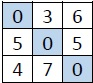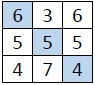# Filling diagonal to make the sum of every row, column and diagonal equal of 3×3 matrix using c++

Suppose we have one 3x3 matrix, whose diagonal elements are empty at first. We have to fill the diagonal such that the sum of a row, column and diagonal will be the same. Suppose a matrix is like −After filling, it will be −Suppose the diagonal elements are x, y, z. The values will be −

• x = (M[2, 3] + M[3, 2])/ 2
• z = (M[1, 2] + M[2, 1])/ 2
• y = (x + z)/2

## Example

Live Demo

#include<iostream>
using namespace std;
void displayMatrix(int matrix) {
for (int i = 0; i < 3; i++) {
for (int j = 0; j < 3; j++)
cout << matrix[i][j] << " ";
cout << endl;
}
}
void fillDiagonal(int matrix) {
matrix = (matrix + matrix) / 2;
matrix = (matrix + matrix) / 2;
matrix = (matrix + matrix) / 2;
cout << "Final Matrix" << endl;
displayMatrix(matrix);
}
int main() {
int matrix = {
{ 0, 3, 6 },
{ 5, 0, 5 },
{ 4, 7, 0 }};
cout << "Given Matrix" << endl;
displayMatrix(matrix);
fillDiagonal(matrix);
}

## Output

Given Matrix
0 3 6
5 0 5
4 7 0
Final Matrix
6 3 6
5 5 5
4 7 4